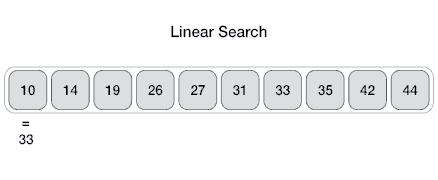Options
All
• Public
• Public/Protected
• All

# External module search

## Type aliases

### IndexOfFn

IndexOfFn: function

IndexOf function type for search algorithms

#### Type declaration

• (collection: T[], value: T, comparator: ComparatorFn<T>): number

## Functions

### binaryIndexOf

• binaryIndexOf<T>(collection: T[], v: T, comparator?: ComparatorFn<T>): number
• Binary search works on sorted arrays. Binary search begins by comparing an element in the middle of the array with the target value. If the target value matches the element, its position in the array is returned. If the target value is less than the element, the search continues in the lower half of the array. If the target value is greater than the element, the search continues in the upper half of the array. By doing this, the algorithm eliminates the half in which the target value cannot lie in each iteration.source: www.mathwarehouse.com

### Complexity

Case BigO
Worst-case performance `O(log n)`
Best-case performance `O(1)`
Average performance `O(log n)`
Worst-case space complexity `O(1)`

### Reference

``````import { binaryIndexOf } from '@pencroff/ts-algorithms/dist/algorithm/search/binary';
const res = binaryIndexOf([3, 5, 8], 8); // 2``````
typeparam

of types T

#### Parameters

• ##### v: T

searching value

### linearIndexOf

• linearIndexOf<T>(collection: T[], v: T, comparator?: ComparatorFn<T>): number
• A linear search sequentially checks each element of the list until it finds an element that matches the target value. If the algorithm reaches the end of the list, the search terminates unsuccessfully.source: www.tutorialspoint.com

### Complexity

Case BigO
Worst-case performance `O(n)`
Best-case performance `O(1)`
Average performance `O(n)`
Worst-case space complexity `O(1) iterative`

### Reference

``````import { linearIndexOf } from '@pencroff/ts-algorithms/dist/algorithm/search/linear';
const res = linearIndexOf([11, 5, 8], 8); // 2``````
typeparam

of types T

#### Parameters

• ##### v: T

searching value